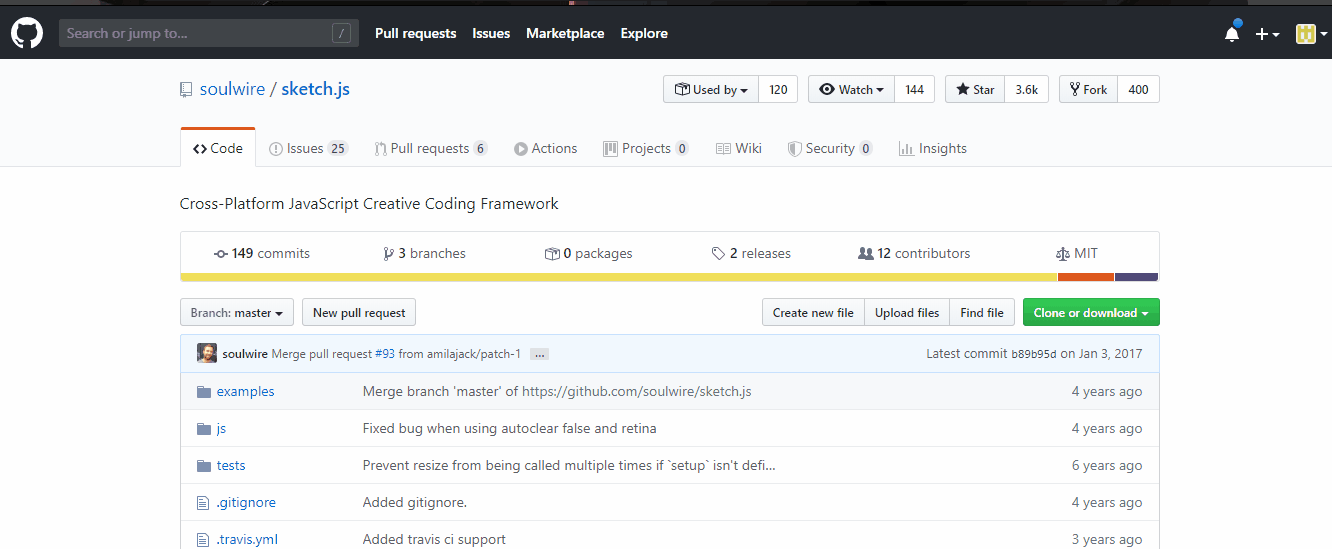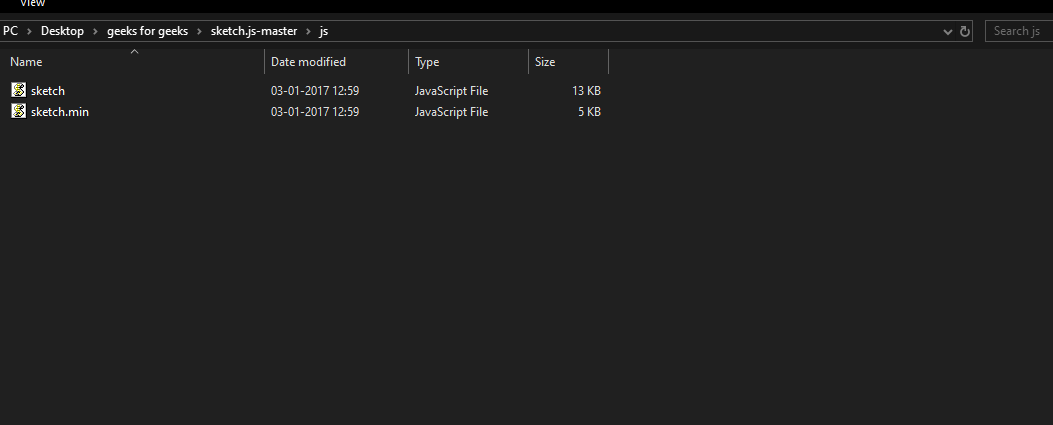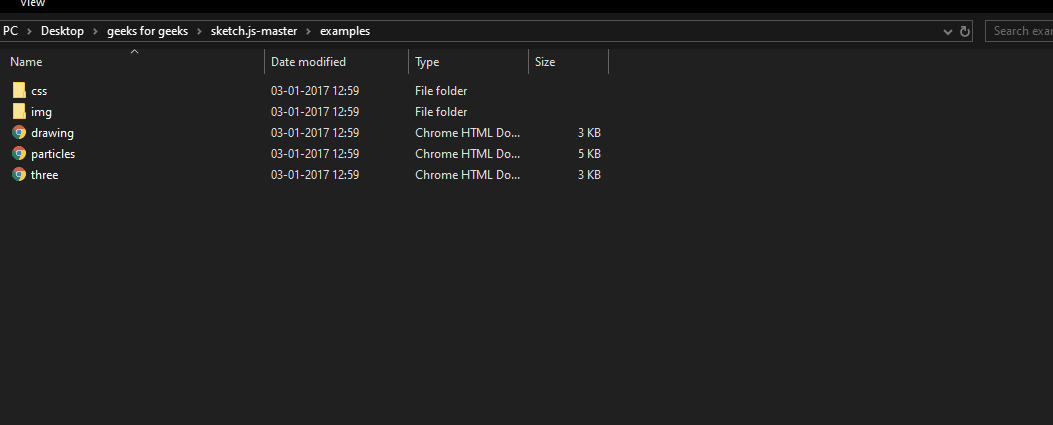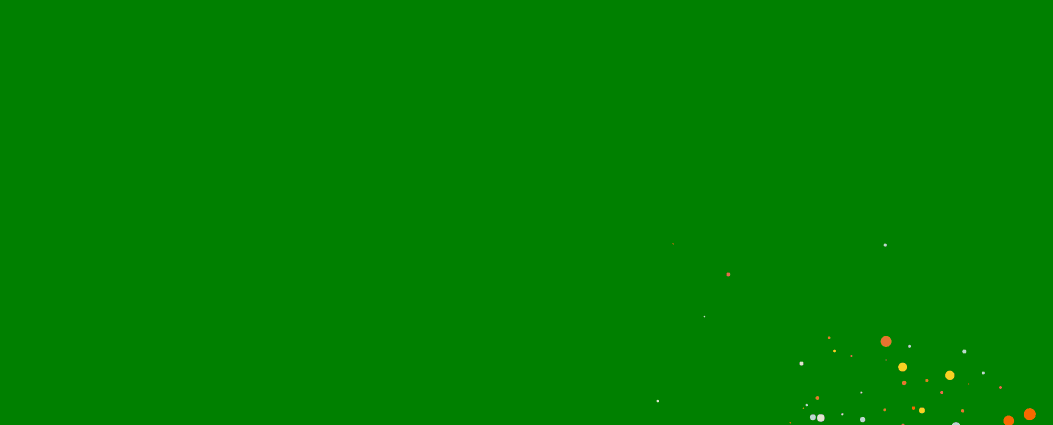# Sketch.js | Introduction

Sketch.js is a light-weight creative coding platform. It provides all the snippets and code that you need to add to make cool animations and effects to your website. Sketch.js makes the implementation of animations very fast and easy. It is similar to using Processing . Its main concept is based on events like mouse events, touch events, and keyboard events. All the events are augmented for convenience so that you can use them easily and outside event handlers if you want to. For getting coordinates of mouse and touch event, we have x and y coordinates which are also used in normal JavaScript. Using sketch.js makes coding faster and fun without worrying about the setup and writing a lot of boilerplate code for the configuration. It provides a built-in graphics context and animation loops. So at last one should use sketch.js for making animations as it is fast, easy to set up and use and it saves you from writing a lot of code.

Installation:• Step-2: Now, extract the zip file. You need to add sketch.min.js file into your project.• Step-3: Now, if you go into the examples folder, you will see three HTML files. All you need to do is to copy the JS of the file whose effect you want to add into your project.• And that’s all the work that you need to do. Below is the final code after adding one of the example’s JS into your project.

Example:

 ` ` `<``html` `lang``=``"en"``> ` ` `  `<``head``> ` `    ``<``meta` `charset``=``"UTF-8"``> ` `    ``<``meta` `name``=``"viewport"` `content``= ` `        ``"width=device-width, initial-scale=1.0"``> ` ` `  `    ``<``title``>Sketch.js ` ` `  `    ``<``style``> ` `        ``body { ` `            ``margin: 0; ` `            ``padding: 0; ` `            ``background: green; ` `        ``} ` `    `` ` ` ` ` `  `<``body``> ` `    ``<``script` `src``=``"sketch.min.js"``> ` `    ``<``script``> ` ` `  `        ``// Particle function ` `        ``function Particle(x, y, radius) { ` `            ``this.init(x, y, radius); ` `        ``} ` ` `  `        ``Particle.prototype = { ` `            ``init: function (x, y, radius) { ` `                ``this.alive = true; ` `                ``this.radius = radius || 10; ` `                ``this.wander = 0.15; ` `                ``this.theta = random(TWO_PI); ` `                ``this.drag = 0.92; ` `                ``this.color = '#fff'; ` `                ``this.x = x || 0.0; ` `                ``this.y = y || 0.0; ` `                ``this.vx = 0.0; ` `                ``this.vy = 0.0; ` `            ``}, ` ` `  `            ``move: function () { ` `                ``this.x += this.vx; ` `                ``this.y += this.vy; ` `                ``this.vx *= this.drag; ` `                ``this.vy *= this.drag; ` `                ``this.theta += random(-0.5, 0.5) * this.wander; ` `                ``this.vx += sin(this.theta) * 0.1; ` `                ``this.vy += cos(this.theta) * 0.1; ` `                ``this.radius *= 0.96; ` `                ``this.alive = this.radius > 0.5; ` `            ``}, ` ` `  `            ``draw: function (ctx) { ` `                ``ctx.beginPath(); ` `                ``ctx.arc(this.x, this.y, this.radius, 0, TWO_PI); ` `                ``ctx.fillStyle = this.color; ` `                ``ctx.fill(); ` `            ``} ` `        ``}; ` ` `  `        ``var MAX_PARTICLES = 280; ` `        ``var COLOURS = ['#69D2E7', ` `            ``'#A7DBD8', ` `            ``'#E0E4CC', ` `            ``'#F38630', ` `            ``'#FA6900', ` `            ``'#FF4E50', ` `            ``'#F9D423']; ` ` `  `        ``var particles = []; ` `        ``var pool = []; ` `        ``var demo = Sketch.create({ ` `            ``container: document.getElementById('container'), ` `            ``retina: 'auto' ` `        ``}); ` ` `  `        ``demo.setup = function () { ` ` `  `            ``// Set off some initial particles. ` `            ``var i, x, y; ` ` `  `            ``for (i = 0; i < ``20``; i++) { ` `                ``x = (demo.width * 0.5) + random(-100, 100); ` `                ``y = (demo.height * 0.5) + random(-100, 100); ` `                ``demo.spawn(x, y); ` `            ``} ` `        ``}; ` ` `  `        ``demo.spawn` `= ``function` `(x, y) { ` `            ``var particle, theta, force; ` `            ``if (particles.length >= MAX_PARTICLES) ` `                ``pool.push(particles.shift()); ` ` `  `            ``particle = pool.length ? pool.pop() : new Particle(); ` `            ``particle.init(x, y, random(5, 40)); ` ` `  `            ``particle.wander = random(0.5, 2.0); ` `            ``particle.color = random(COLOURS); ` `            ``particle.drag = random(0.9, 0.99); ` ` `  `            ``theta = random(TWO_PI); ` `            ``force = random(2, 8); ` ` `  `            ``particle.vx = sin(theta) * force; ` `            ``particle.vy = cos(theta) * force; ` ` `  `            ``particles.push(particle); ` `        ``}; ` ` `  `        ``demo.update = function () { ` ` `  `            ``var i, particle; ` ` `  `            ``for (i = particles.length - 1; i >= 0; i--) { ` ` `  `                ``particle = particles[i]; ` ` `  `                ``if (particle.alive) particle.move(); ` `                ``else pool.push(particles.splice(i, 1)); ` `            ``} ` `        ``}; ` ` `  `        ``demo.draw = function () { ` ` `  `            ``demo.globalCompositeOperation = 'lighter'; ` ` `  `            ``for (var i = particles.length - 1; i >= 0; i--) { ` `                ``particles[i].draw(demo); ` `            ``} ` `        ``}; ` ` `  `        ``demo.mousemove = function () { ` ` `  `            ``var particle, theta, force, touch, max, i, j, n; ` ` `  `            ``for (i = 0, n = demo.touches.length; i < ``n``; i++) { ` ` `  `                ``touch` `= ``demo``.touches[i], ``max` `= ``random``(1, 4); ` `                ``for (``j` `= ``0``; j < max; j++) { ` `                    ``demo.spawn(touch.x, touch.y); ` `                ``} ` ` `  `            ``} ` `        ``}; ` `    `` ` ` ` ` `  ` `

If we take a look at the above code, all we have done is provide a background add two JS files one is sketch.min.js and other is from one of the files from example folder. We have demonstrated the particle here.

Output:My Personal Notes arrow_drop_upCheck out this Author's contributed articles.

If you like GeeksforGeeks and would like to contribute, you can also write an article using contribute.geeksforgeeks.org or mail your article to contribute@geeksforgeeks.org. See your article appearing on the GeeksforGeeks main page and help other Geeks.

Please Improve this article if you find anything incorrect by clicking on the "Improve Article" button below.

Article Tags :

Be the First to upvote.

Please write to us at contribute@geeksforgeeks.org to report any issue with the above content.